# 2nd PUC Physics Question Bank Chapter 2 Electrostatic Potential and Capacitance

## Karnataka 2nd PUC Physics Question Bank Chapter 2 Electrostatic Potential and Capacitance

### 2nd PUC Physics Electrostatic Potential and Capacitance NCERT Text Book Questions and Answers

Question 1.
Two charges 5 x 10-8 C and -3 x 10-8 C are located 16 cm apart. At what point(s) on the line joining the two charges is the electric potential zero? Take the potential. at infinity to be zero.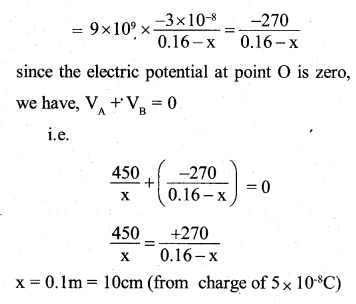Question 2
A regular hexagon of side 10 cm has a charge of 5 µC at each of its vertices. Calculate the potential at the centre of the hexagon.
Let O be the centre of the hexagon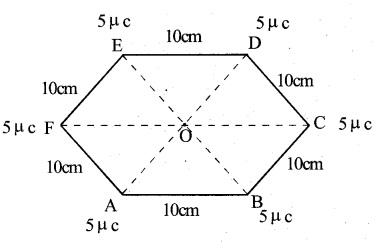It follows that the point O, when joined to the two ends of a side of the hexagon forms an equilateral triangle.
∴ AO = BO = CO = DO = EO = FO = 10 cm = 0.1 m
Since at each comer of the hexagon, a charge of 5pc i.e.5 x 10-6C is placed, the total electric potential at point o due to the charges at the six comers,Question 3
Two charges 2 μC and -2 μC are placed at points A and B 6 cm apart.
(a) Identify an equipotential surface of the system.
(b) What is the direction of the electric field at every point on this surface?(a) The equipotential surface is the plane pependicuIarto the line AB joining the two charges and passing through the mid-point. On this plane, the potential is zero everywhere.
(b) The direction of the electric field is from positive to negative charge i.e. A to B, which is in fact perpendicular to the equipotential plane.

Question 4.
The spherical conductor of a radius of 12 cm has a charge of 1.6 x 10-7C distributed uniformly on its surface. What is the electric field
(a) Inside the sphere
(b) Just outside the sphere
(c) At a point 18 cm from the centre of the sphere?
Here, q = 1.6 x 10-7C.
Radius of the sphere; R = 12cm = 0.12m
(a) Inside the sphere: the charge on a conductor resides on its outer surfaces. Therefore, the electric field inside the sphere is zero.

(b) Just outside the sphere: the charge may be assumed to be concentrated at its centre. Therefore electric field just outside the sphere.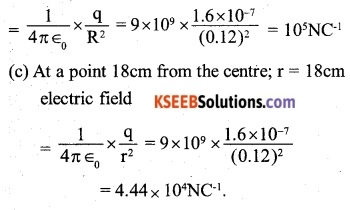Question 5.
A parallel plate capacitor with air between the plates has a capacitance of 8 pF
(1pF = 10-12 F). What will be the capacitance if the distance between the plates is reduced by half, and the space between them is filled with a substance of dielectric constant 6?Question 6.
Three capacitors each of capacitance 9 pF are connected in series.
(a) What is the total capacitance of the combination?
(b) What is the potential difference across each capacitor if the combination is connected to a 120 V supply?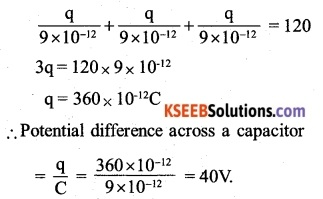Question 7.
Three capacitors of capacitances 2 pF, 3 pF, and 4 pF are connected in parallel.
(a) What is the total capacitance of the combination?
(b) Determine the charge on each capacitor if the combination is connected to a 100 V supply.Question 8.
In a parallel plate capacitor with air between the plates, each plate has an area of 6 x 10-3 m2 and the distance between the plates is 3 mm. Calculate the capacitance of the capacitor. If this capacitor is connected to a 100 V supply, what is the charge on each plate of the capacitor?When the capacitor is connected to a 100V supply, the charge on each plate of the capacitor,
q = CV = 1.77 x 10-11 x 100 = 1.77 x 109C.

Question 9.
Explain what would happen if, in the capacitor given in Exercise 2.8, a 3 mm thick mica sheet (of dielectric constant = 6) were inserted between the plates,
(a) while the voltage supply remained connected.
(b) after the supply was disconnected.
(a) When the voltage supply connected The capacitance of the capacitor will become k times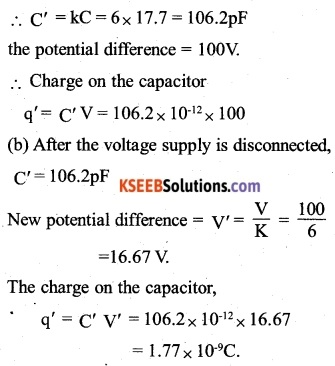Question 10.
12pF capacitor is connected to a 50V battery. How much electrostatic energy is stored in the capacitor?Question 11.
A 600pF capacitor is charged by a 200V supply. It is then disconnected from the supply and is connected to another uncharged 600 pF capacitor. How much electrostatic energy is lost in the process?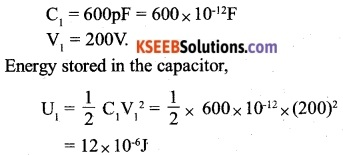When this charged capacitor is connected to another uncharged capacitor C2 (= 600 pF), they will share charges till the potential differences across their plates become equal.
The total charge on the two capacitors,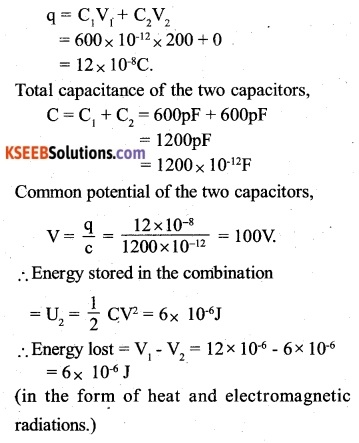Question 12.
A charge of 8 mC is located at the origin. Calculate the work done in taking a small charge of -2 ×10-9 C from a point P (0,0, 3 cm) to a point Q (0, 4 cm, 0), via a point R (0, 6 cm, 9 cm).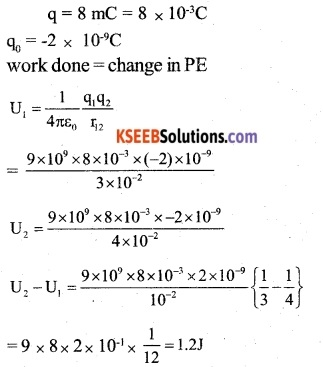Question 13.
A cube of side ‘b’ has a charge ‘q’ at each of its vertices. Determine the potential and electric field due to this charge array at the centre of the cube.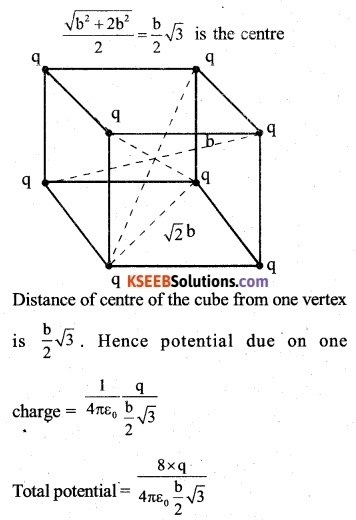The field at the centre of the cube due to the charge at the opposite vertices cancels each other. Hence net fields as the centre of the cube are zero.Question 14.
Two tiny spheres carrying charges 1.5 µC and 2,5 µC are located 30 cm apart. Find the potential and electric field
(a) at the midpoint of the line joining the two charges, and
(b) at a point 10 cm from this midpoint in a plane normal to the line and passing through the midpoint.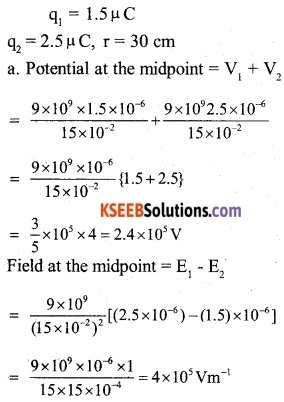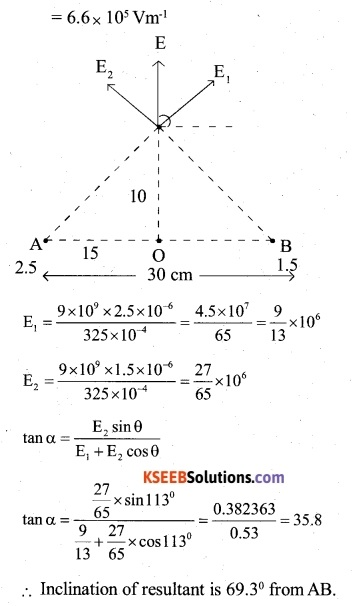Question 15.
The spherical conducting shell of inner radius r1 ( and outer radius r2 has a charge Q.
(a) A charge ‘q’ is placed at the centre of the shell. What is the surface charge density on the inner and outer surfaces of the shell?
(b) Is the electric field inside a cavity (with no charge) zero, even if the shell is not spherical, but has an irregular shape? Explain.Question 16.
(a) Show that the normal component of electrostatic field has a discontinuity from one side Of a charged surface to another given by (E2 -E1), $$\hat { n }$$ = $$\frac { \sigma }{ { \varepsilon }_{ 0 } }$$ ? where is a unit vector normal to the surface at a point and a is the surface charge density at that point (The direction of ft if from side 1 to 2.) Hence show that just outside a conductor, the electric field is

(b) Show that the tangential component of the electrostatic field is continuous from one side of a charged surface to another [Hint: For (a), use Gauss law, For, (b) use the fact that work done by electrostatic field on a closed loop is zero.]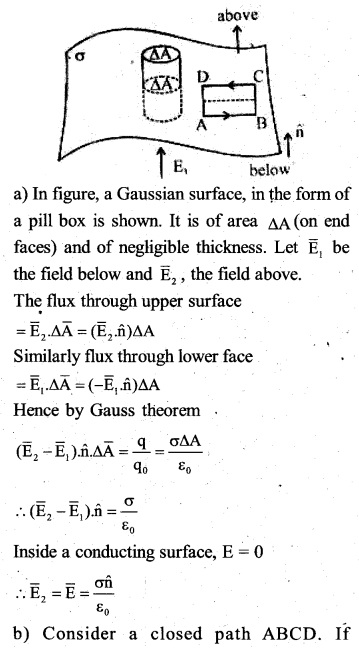Question 17.
A long charged cylinder of linear charge density x is surrounded by a hollow coaxial conducting cylinder. What is the electric field in the space between the two cylinders?
Charge density = λ
The flux calculated for the cylindrical surface of radius r, over a length 1 is E.2 πrl.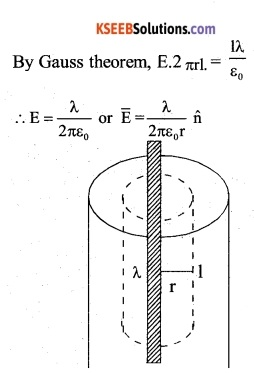Question 18.
In a hydrogen atom, the electron and proton are bound at a distance of about 0.533 A.
(a) Estimate the potential energy of the system in eV, taking the zero of the potential energy at an infinite separation of the electron from the proton.
(b) What is the minimum work required to free the electron? Given that its kinetic energy in the orbit is half the magnitude of potential energy obtained in (a).
(c) What are the answers to (a) and (b) above if the zero of potential energy is taken at 1.06 separation?
r = 0.53 A = 0.53 x 1010m
Charge of electron = -e
Charge of proton = e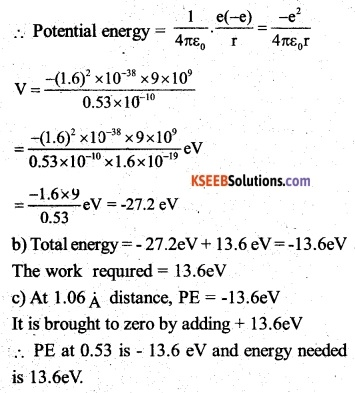(b)Total energy = -27.2eV+ 13.6 eV=-13.6eV The work required 13.6eV
(c) At 1.06 A distance, PE = -13.6eV
It is brought to zero by adding + 13.6eV
∴ PE at 0.53 is – 13.6 eV and energy needed is 13.6eV.

Question 19.
If one of the two electrons of an H2 molecule is removed, we get a hydrogen molecular ion H2+. In the ground state of an H2+ the two protons are separated by roughly  1.5 A, and the electron is roughly 1 A from each proton. Determine the potential energy of the system. Specify your choice of zero potential energy.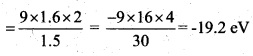Question 20.
Two charged conducting spheres of radii a and b are connected to each other by a wire. What is the ratio of electric fields at the surfaces of the two spheres? Use the result obtained to explain why the charge density on the sharp and pointed ends of a conductor is higher than on its flatter portions.As A → 0 E becomes extremely large.
Flat means A → ∞, hence E is very small.Question 21.
Two charges – q and + q are located at points (0,0, -a) and (0, 0, a), respectively.
(a) What is the electrostatic potential at the points (0,.0,z) and (x,y,0)?
(b) Obtain the dependence of potential on the distance r of a point from the origin
$$\frac { r }{ a } >>1$$
(c) How much work is done in moving a small test charge from the point (5,0,0) to
(-7, 0, 0) along the x-axis? Does the answer change if the path of the test charge between the same points is not along the x-axis?
(a) The configuration is  similar to a dipole;(b) The dependence on r is as $$\frac { 1 }{ { r }^{ 2 } }$$

(c) Zero No, because work done by electrostatic field between two points is independent of the path connecting the two points.

Question 22.
The figure shows a charge array known as an electric quadrupole. For a point on the axis of the quadrupole, obtain the dependence of potential on ‘r’ for $$\frac { r }{ a } >>1$$, a and contrast your results with that due to an electric dipole, and an electric monopole (i.e., a single charge).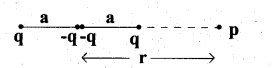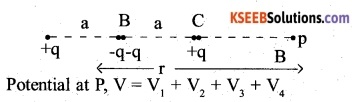Question 23.
An electrical technician requires a capacitance of 2 pF in a Circuit across a potential difference of 1 kV. A large number oflgFcapacitors are available to him each of which can withstand a potential difference of not more than 400 Y. Suggest a possible arrangement that requires the minimum number of capacitors.Question 24.
What is the area of the plates of a 2F parallel plate capacitor, given that the separation between the plates is 0.5 cm? [You will realise from your answer why ordinary capacitors are in the range of µF or less. However, electrolytic capacitors do have a much larger capacitance (0.1F) because of the very minute separation between the conductors.]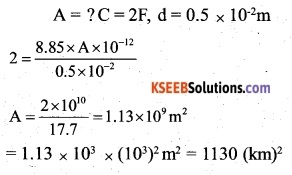Question 25.
Obtain the equivalent capacitance of the network in figure below. For a 300 Y supply, determine the charge and voltage across each capacitor.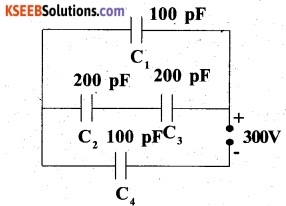Question 26.
The plates of a parallel plate capacitor have an area of 90 cm2 each and are separated by 2.5 mm. The capacitor is charged by connecting it to a 400 V supply.
(a) How much electrostatic energy is stored by the capacitor?
(b) View this energy as stored in the electrostatic field between the plates, and obtain the energy per unit volume u. Hence arrive at a relation between u and the magnitude of electric field E between the plates.
A= 90 cm2 = 90 x 10-4 m2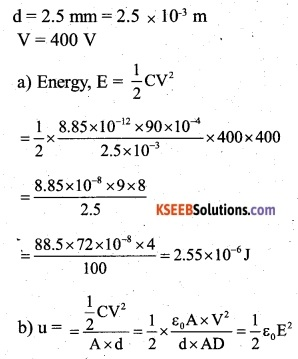Question 27.
A 4µF capacitor is charged by a 200 V supply. It is then disconnected from the supply and is connected to another uncharged 2pF capacitor. How much electrostatic energy of the first capacitor is lost in the form of heat and electromagnetic radiation?Question 28.
Show that the force on each plate of a parallel plate capacitor has a magnitude equal to $$\left( \frac { 1 }{ 2 } \right)$$ QE, where Q is the charge on the capacitor, and E is the magnitude of electric field between the plates. Explain the origin of the factor $$\left( \frac { 1 }{ 2 } \right)$$.The physical origin of the factor $$\frac { 1 }{ 2 }$$ in the force formula lies in the fact that just outside the conductor, the field is E, and inside it is zero. So the average value $$\frac { E }{ 2 }$$ contributes to the force.

Question 29.
The spherical capacitor has an inner sphere of radius 12 cm and an outer sphere of radius 13 cm. The outer sphere is earthed and the inner sphere is given a charge of 2.5 µ C. The space between the concentric spheres is filled with a liquid of dielectric constant 32.
(a) Determine the capacitance of the capacitor.
(b) What is the potential of the inner sphere?
(c) Compare the capacitance of this capacitor with that of an isolated sphere of radius 12cm. Explain why the latter is much smaller.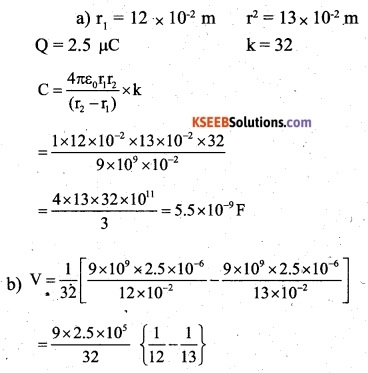Question 30.
(a) Two large conducting spheres carrying charges Q1 and Q2 are brought close to each other. Is the magnitude of electrostatic force between them exactly given by $$\frac { { Q }_{ 1 }{ Q }_{ 2 } }{ 4\pi { \varepsilon }_{ 0 }{ r }^{ 2 } }$$, where r is the distance between their centres?
(b) If Coulomb’s law involved $$\frac { 1 }{ { r }^{ 3 } }$$. (instead 0f $$\frac { 1 }{ { r }^{ 2 } }$$),
Gauss law be still true?
(c) A small test charge is released at rest at a point in an electrostatic field configuration. Will it travel along the field line passing through that point?
(d) What is the work done by the field of a nucleus incomplete circular orbit of the electron? What if the orbit is elliptical?
(e) We know that the electric field is discontinuous across the surface of a charged conductor. Is electric potential also discontinuous there?
What meaning would you give to the capacitance of a single Conductor?
(f) Guess a possible reason why water has a much greater dielectric constant (= 80) than say, mica (= 6).
(a) No, because the distribution of charge on the conducting spheres becomes non-uniform when they are brought closer to each other. Coulomb’s law is valid for point charges only or for spheres on which charge distribution is uniform.
(b) If dependence of Coulomb’s law involves 1/r3 dependence then Gauss’s law in the existing state will not be true.
(c) It is true only if the field line is a straight line.
(d) Whatever may be the shape of the closed orbit, it is zero.
(e) Potential is continuous.
(f) It means that a single conductor is a capacitor whose other plates can be considered to be at infinity.
(g) A water molecule has a permanent dipole moment so its dielectric constant is high. Mica does not have polar molecules.Question 31.
A cylindrical capacitor has two co-axial cylinders of length 15 cm and radii 1.5 cm and 1.4 cm. The outer cylinder is earthed and the inner cylinder is given a charge of 3.5 p C. Determine the capacitance of the system and the potential of the inner cylinder. Neglect end effects (i.e., bending of field lines at the ends)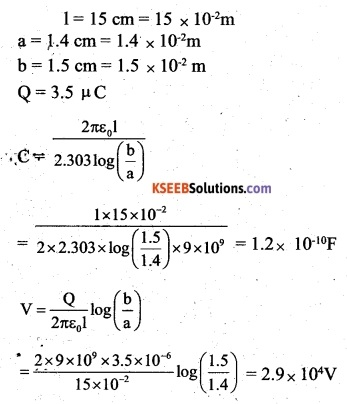Question 32.
A parallel plate capacitor is to be designed with a voltage rating of 1kV, using a material of dielectric constant 3 and dielectric strength of about 107 Vm-1. (Dielectric strength is the maximum electric field a material can tolerate without breakdown,i.e  without starting to conduct electricity through partial ionisation.) For safety, we .should like the field never to exceed, say 10% of the dielectric strength. What minimum area of the plates is required to have a capacitance of 50 pF?Question 33.
Describe schematically the equipotential surfaces corresponding to
(a) A constant electric field in the z-direction
(b) A field that uniformly increases in magnitude but remains in a constant (say, z) direction
(c) A single positive charge at the origin and a uniform grid consisting of long equally spaced parallel charged wired in a plane.
(a) Equally spaced x-y planes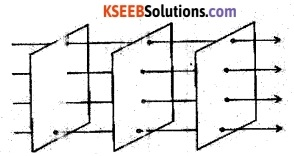(b) x – y planes getting closer and closer along the z-direction.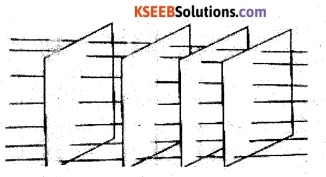(c) Concentric spherical surface gradually increasing in spacing(d) A periodically varying shape near the grid which gradually reaches the shape of the planes parallel to the grid at far distances.Question 34.
In a van de Graaff-type generator, a spherical metal shell is to be a 15 x 106 V electrode. The dielectric strength of the gas surrounding the electrode is 5 x 107 Vm1. What is the minimum radius of the spherical shell required? (You will learn from this exercise why one cannot build an electrostatic generator using a very small shell which required a small charge to acquire a high potential.)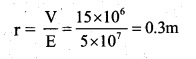Question 35.
A small sphere of radius r1 and charge qt is enclosed by a spherical shell of radius r2 and charge q2. Show that if q2 is positive, the charge will necessarily flow from the sphere to the shell (when the two are connected by a wire) no matter what the charge q2 on the shell is,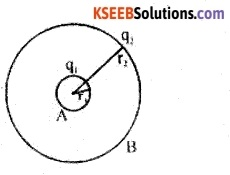The potential inside the shell is constant and is equal to that on its surface. The potential difference then depends only on qr Potential is highest on A. Hence charge flows from A to B irrespective of the value of q2.

Question 36.
(a) The top of the atmosphere is at about 400 kV with respect to the surface of the earth, corresponding to an electric field that decreases with altitude. Near the surface of the earth, the field is about 100 Vmr1. Why then do we not get an electric shock as we step out of our house into the open? (Assume the house to be a steel cage so there is no field inside)

(b) A man fixes outside his house one evening a two-meter high insulating slab carrying on its top a large aluminium sheet of area lm2. Will he get an electric shock if he touches the metal sheet the next morning?

(c) The discharging current in the atmosphere due to the small conductivity of air is known to be 1800 A on average over the globe. Why then does the atmosphere not discharge itself completely in due course and become electrically neutral? In other words, what keeps the atmosphere charged?

(d) What are the forms of energy into which the electrical energy of the atmosphere is dissipated during lightning?
(Hint: The earth has an electric field of about 100 Vm1 at its surface in the downward direction, corresponding to a surface charge density = -10-9 Cm-2. Due to the slight conductivity of the atmosphere upto about 50 km (beyond which it is a good conductor), about + 1800 C is pumped every second into the earth as a whole. The earth, however, does hot get discharged since thunderstorms and lightning occurring continually all over the globe pump an equal amount of negative charge on the earth.)
(a) Our body and the earth’s surface become equipotential (at equal potential). It means there is no potential difference between the earth and our body. Hence no current flows through our body and therefore we do not experience an electric shock.

(b) The aluminum sheet and the earth form a capacitor with the insulating slab as the dielectric. The downpour of the atmospheric charge will raise the potential of the sheet of aluminium. When we touch the aluminium sheet, the charge will flow to the earth through our bodies. This flow of charge constitutes an electric current and we will experience a shock.

(c) No doubt the atmosphere continuously gets charged due to lightning, thunderstorms but simultaneously it gets discharged through normal weather zones. This keeps the system balanced.

(d) Electrical energy of the atmosphere appears as light, sound, and heat energies during thunderstorms and lightning.

### 2nd PUC Physics Electrostatic Potential and Capacitance Entrance Examination Questions and Answers

Question 1.
On moving a charge of 20C by 2cm, 2J of work is done. Then, the potential difference between the points is: (AIEEE 2002)
(A) 1 V
(B) 8V
(C) 2V
(D) 0.5V.
(A) 1 V

Question 2.
Two points P and Q are maintained at potentials 10V and -4V respectively. The work done in moving 100 electrons from P to Q is …………………… (AIEEE 2009)
(A) -9.60 x 10-17J
(B) 9.60 x 10-17J
(C)-2.04 x 10-16J
(D) 2.24 × 10-16J
(D) 2.24 × 10-16J

Question 3.
Capacitance (in F) of a spherical conductor of radius 1m is: (AIEEE 2005)
(A) 1.1 x 10-10J
(B) 10-6
(C) 9 ×109J
(D) 103
(A) 1.1 x 10-10J

Question 4.
If there are ‘n’ capacitors in parallel connected to V volt source, then the energy stored is equal to (AIEEE 2002)
(A) CV
(B) $$({ 1 }/{ 2 }C{ V }^{ 2 })n$$
(C) CV2
(D) $${ 1 }/{ 2 }C{ V }^{ 2 }$$
(B) (Kcv)n

Question 5.
The unit of permittivity of free space (ε0) is   (CBSE 2004)
(A) CN-1 m-1
(B) Nm2C-2
(C) C2N2 m2
(D) C2N 2m 2
(C)

Question 6.
The electric potential at a point in free space due to charge Q coulomb is Q x 1011 volts. The electric field at that point is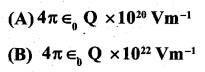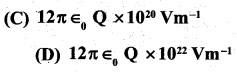Question 7.
In bringing an electron towards another electron, the electrostatic potential energy of the system : (CBSE 1999)
(A) Increase
(B) Decreases
(C) Remains unchanged
(D) Becomes zero
(A) Increase

Question 8.
What is the effective capacitance between X and Y? (CBSE 2001, AIEEE 1998)
(A) 24 pF
(B) 18 pF
(C) 12 pF
(D) 6pF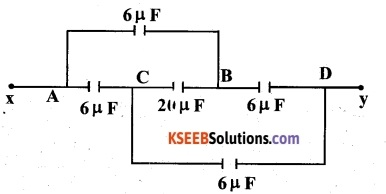The dimensions of $$\frac { 1 }{ 2 } { \varepsilon }_{ 0 }{ E }^{ 2 }$$ where $${ \epsilon }_{ 0 }$$ is permittivity of free space and E is electric field is  (CBSE 2010)